# Graphs and networks

## Intro

This site contains the description of the course Graphs and Networks (NDMI110) organized primarily for students of the second grade of the Bachelor's Computer Science program in Czech as well as English versions. This course is one of the Suggested Elective courses (Povinně volitelné in Czech) for specialization General Computer Science, see description in Czech or English.

## Description

Many real-world systems possess a natural network structure. Examples include social systems such as Facebook, Twitter, or real-world communities, biological systems such as the human brain, protein interaction systems, or metabolic networks, technological systems such as the Internet, or World-Wide-Web, financial systems such as stock markets, or trade networks, and many others. We can often provide much information about these systems using analysis of the corresponding networks, such as characterizing Alzheimer's disease effects, financial crisis structure, construction of autonomous recommender systems, finding bottlenecks of computer networks, or predicting the effects of the newly constructed drugs. This course aims to introduce such a vast area of complex network theory concentrated on graph-theoretic properties of these networks and corresponding algorithmic solutions.

This course is interesting either to students searching for combinatorial and graph-theoretic properties and problems dealing with recent open problems enable to solve real-world situations or to students aiming to study machine learning and artificial intelligence where these networks find the huge application, e.g. through emerging notions such as graph neural network.

The course is designed for students in the second grade of the Bachelor's Computer Science program and aims to explain many notions already discussed in other courses from a new perspective, also providing new properties, problems, and results, see also context of the course. All notions will be either redefined or reminded sufficiently. These notions include elements from courses of Discrete mathematics, Linear algebra, Probability and statistics, and Algorithms and Data Structures. For notions reused, we often provide a new perspective and utilization.

There is a consequent course dealing with advanced topics of complex networks from a graph theory perspective for students interested in the topic, see corresponding site or SIS reference as Complex network analysis (NDMI096).

## Topics

The course consists of the following topics. For the context, see the section below.

1. Introduction to complex networks
• Intruduction to area of complex networks
• Application examples such as social network, Inet, WWW, protein-protein
• Description of basic notions and phenomena such as scale-free, small-world, community structure, etc.
2. Characteristic structure of a complex network
• Simplified Scale-free (SF) property generalizing degree sequence
• Simplified small-world (SW) property generalizing reachability and local density
• Simplified community structure (CS) generalizing cliques of graphs
3. Network centrality
• Network centrality generalizing vertex degree, distinguising local and global centrality
• Centralities based on distances generalizing eccentricity such as closeness centrality
• Betweenness centrality and its combinatorial properties
4. Computations of centralities
• Comutation of Betweenness centrality (BC) and issues for large graphs.
• Algorithm of Brandes for Betweeneess centrality.
• Some bounds for Betweenness centrality simplifying its computations.
5. Combinatorial properties of centralities
• General bounds for betweeness centrality..
• Effects on adding/removing vertices/edges on BC.
• Graphs with global conditions on centralities such as betweenness uniform graphs.
6. Eigenvector centrality
• Introduction to basic necessary notions of spectral graph theory.
• Definition of eigenvector centrality as generalization of degree centrality.
• Introduction of generalizations such as PageRank.
7. Introduction to random graph
• Recap or introduction of notions from probability and statistics.
• Definition of basic Erdos-Renyi (ER) graph.
• Basic properties of ER graph including emmergence of a giant component.
8. Properties of random graphs
• Definition of properties of random graph through a limiting property.
• Basic properties of ER model.
• Definition of real-world graphs properties such as Small-world or scale-free.
9. Real-world random graphs
• Requirements for real-world random networks.
• Introduction of degree preservning models such as configuration model (CM) and its properties.
• Introduction of network growing models such as Barabasi-Albert (BA) model ans its propertis.
10. Community structure
• Introduction to community structure (CS) for a graph and community detection (CD) problem.
• Community structure as MAX-CUT problem and consequent algorithmic complexity.
• Hierarchical approach to community and Girvan-Newman algorithm.
11. Evaluating community using modularity
• Introduction of modularity measure and its utilization in Girvan-Newman algorithm.
• Maximization of modularity and basic bounds for this measure.
• Anti-community structure and minimal modularity values.
12. Processes on networks
• General definition of a process on a network.
• Example os SIR model for epidemic spreading.
• Properties of network processes and their stability.
13. Extended topics (it time allows)
• Network motifs as representants of frequent subgraphs in complex netwoprks.
• Percolation theory representing suddent change in complex networks.
• Graphlets as small subgraphs generalizing neighborhood.

## Context

The following chapter provides the context of the course in the whole Bachelor's Computer Science program. The following mindmap contains a deeper description of the lectures along with connections to other preceding courses. List of topics is also given above in a separe section.

Note as

## Organization

The course is organized in lectures providing basic theory on topics given above. Seminars are divided into two groups:

1. Seminars providing theoretical exercises making students more familiar with the theory,
2. practical seminars where some phenomena will be tested on real-world networks.

Continuous evaluation is based on combination of test and individual work based on theoretical or practical problems. Final evaluation consists of combined written and oral exam.

This section contains the list of references basic and advanced for the course, some of which are available online..

#### Basic references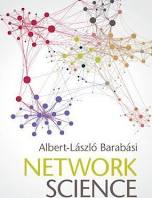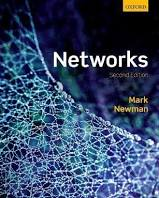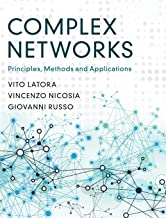Albert-Laszco Barabasi MEJ Newmann V. Latora, V. Nicosia, G. Russo
Network Science Networks: an introduction Complex networks
Cambridge University Press, 2016 Oxford University Press, 2018 Cambridge University Press, 2017

#### Extended references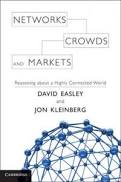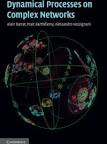D. Easly, J. Kleinberg A. Barrat, M. Barthelemy, A. Vespignani
Networks, Crowds and Markets Dynamical Processes on Complex Networks
Cambridge University Press, 2010 Cambridge University Press, 2012